|

# 速威MES解决方案 引领耀东华智能工厂产业升级

2018年1月18日，速威MES解决方案广东耀东华智能工厂项目启动大会在广东佛山耀东华总部隆重举行。中国领先的MES解决方案供应商广州速威智能系统科技有限公司，携手国家高新技术企业、广东省林业龙头企业耀东华公司，共同打造中国智造2025在林业产业、智装行业、装饰材料行业的标杆企业。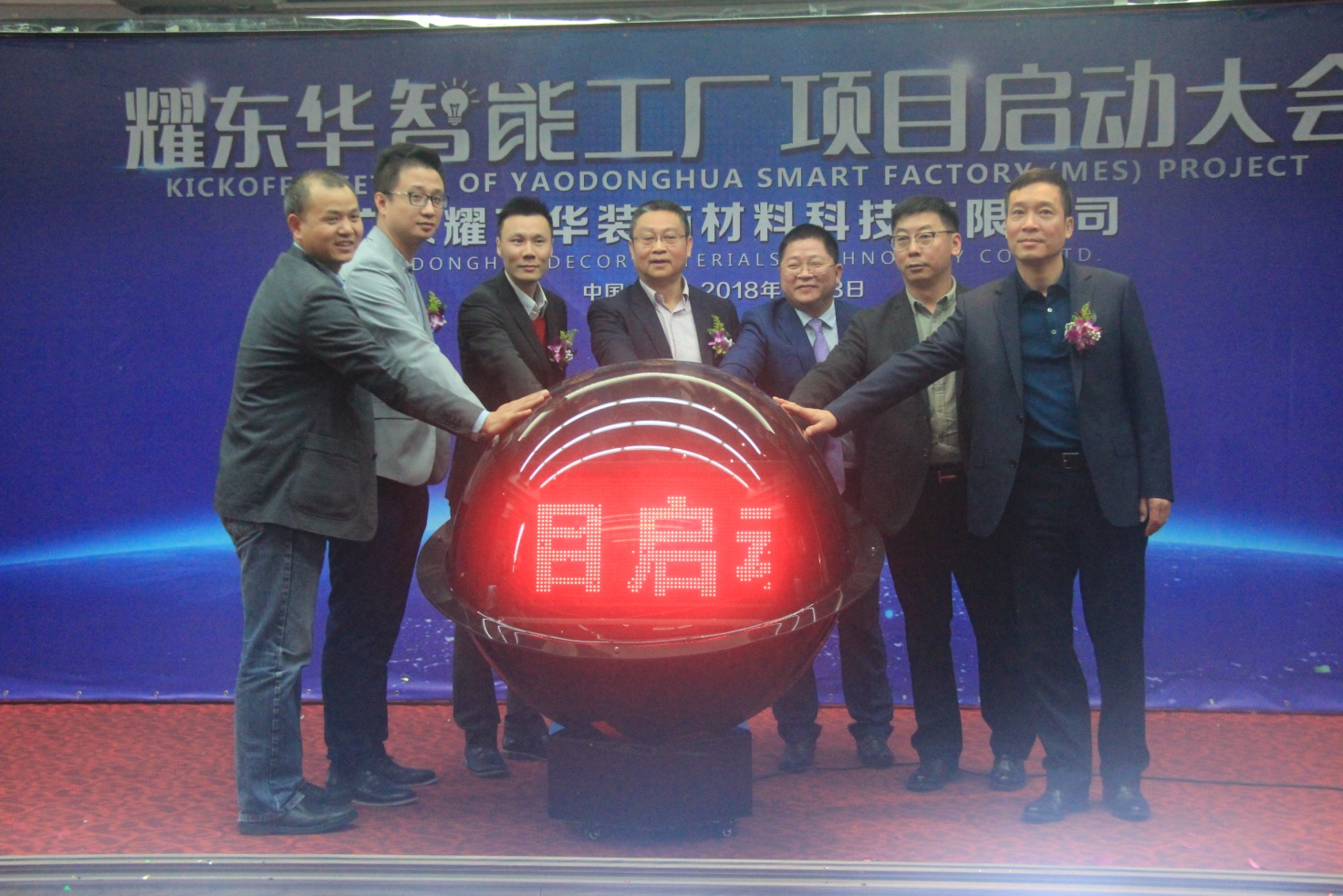主礼嘉宾共同启动耀东华智能工厂项目

佛山市南海区里水镇领导班子成员、党委委员谢永欣、南海经贸局技改科科长吴其奋、里水镇经促局局长梁敬华、里水镇经促局副局长林中强、华南农业大学材料与能源学院副院长胡传双、佛山市高新技术产业协会秘书长陈伟成、广东省家具商会执行会长兼秘书长、广东省家具产业研究院院长蒋德辉、中国智能化装饰专业委员会专家组副组长兼实验室主任、耀东华高级顾问项敏、广州速威智能系统科技有限公司董事长郭孔快、广东耀东华装饰材料科技有限公司董事长曾敏华、以及广东用友公司制造事业部总经理王浪峰等重要嘉宾出席了启动大会。

南海区里水镇领导班子成员、党委委员谢永欣指出，鼓励推动企业智能制造、科技创新的转型升级一直是我们倡导的，此次耀东华智能工厂项目的启动，推动了工业互联网、云计算、大数据和生产制造的深度融合，必将为地域产业的发展注入新活力，并代表镇政府祝愿耀东华智能工厂项目启动大会取得圆满成功。

耀东华董事长曾敏华表示，耀东华立志打造成为中国最好的智能制造饰面人造板企业，对智能制造水平十分重视。今天，在各位贵宾的见证下，速威MES解决方案项目正式启动。这次引进MES制造执行系统和NC云技术企业管理系统，提升智能精益制造及数字管理水平，彰显了耀东华转型升级进入智能制造时代的决心。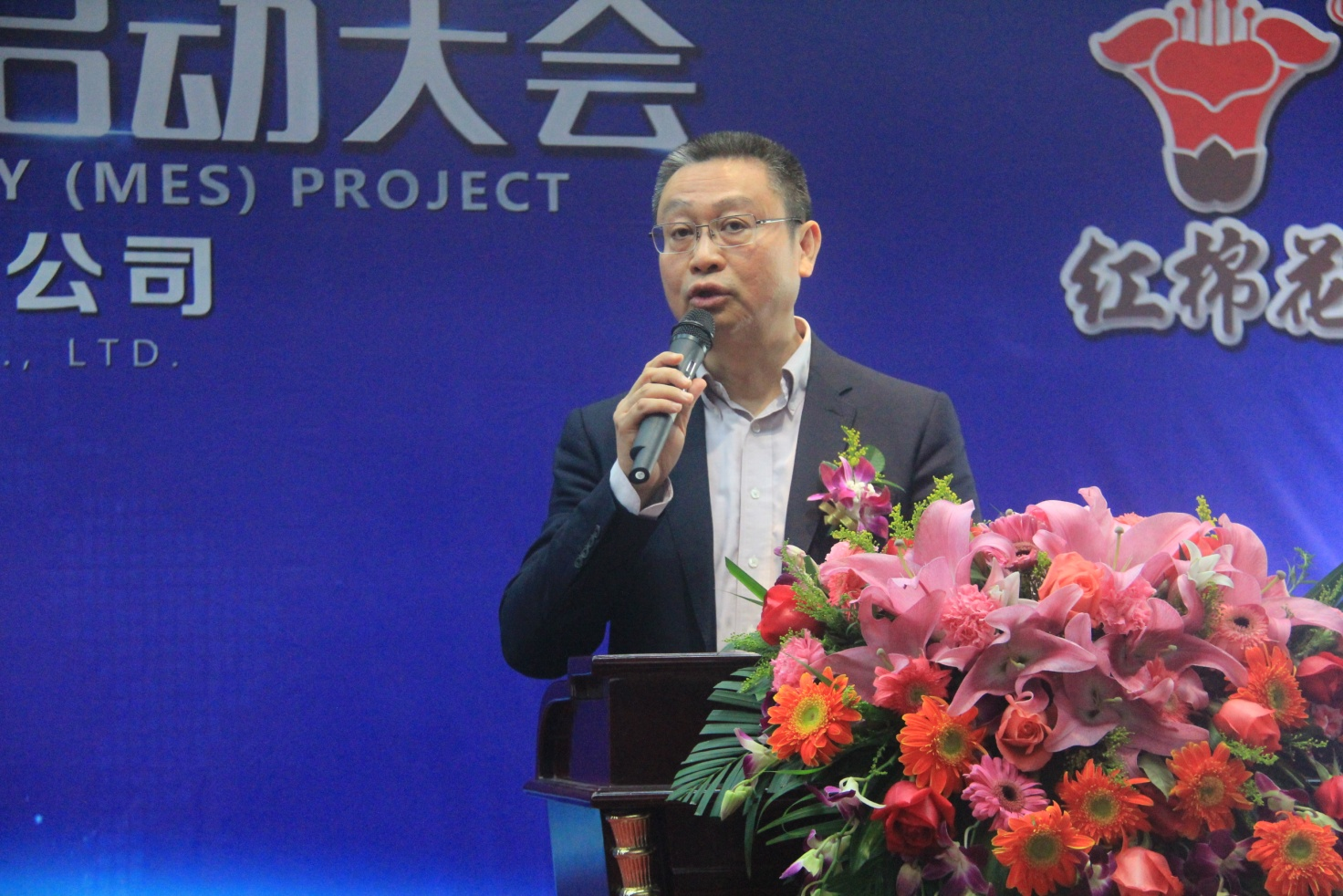耀东华董事长曾敏华致辞

据悉，MES（Manufacturing Execution System），中文名称为“制造执行系统“，是用来帮助企业从接获订单、进行生产、流程控制一直到产品完成，主动收集及监控制造过程中所产生的生产资料，以确保产品生产质量的应用软件。

广东耀东华智能工厂是国际领先的数字化、信息化工厂，通过建立MES制造执行系统，实现计划、调度、质量、设备、生产、能效等管理功能，从最初的原材料进料到最后的成品仓储物流，所有环节均由机器设备自动完成，先进精准。再结合ERP企业资源计划系统和NC系统所实现的供应链、物流、成本、商品管理等经营管理功能，完美融合智能生产+智慧物流，实现数字化控制状态、信息实时监测和自动适应控制，提高产品全生命周期管理、客户关系管理、供应链管理系统的智能化水平。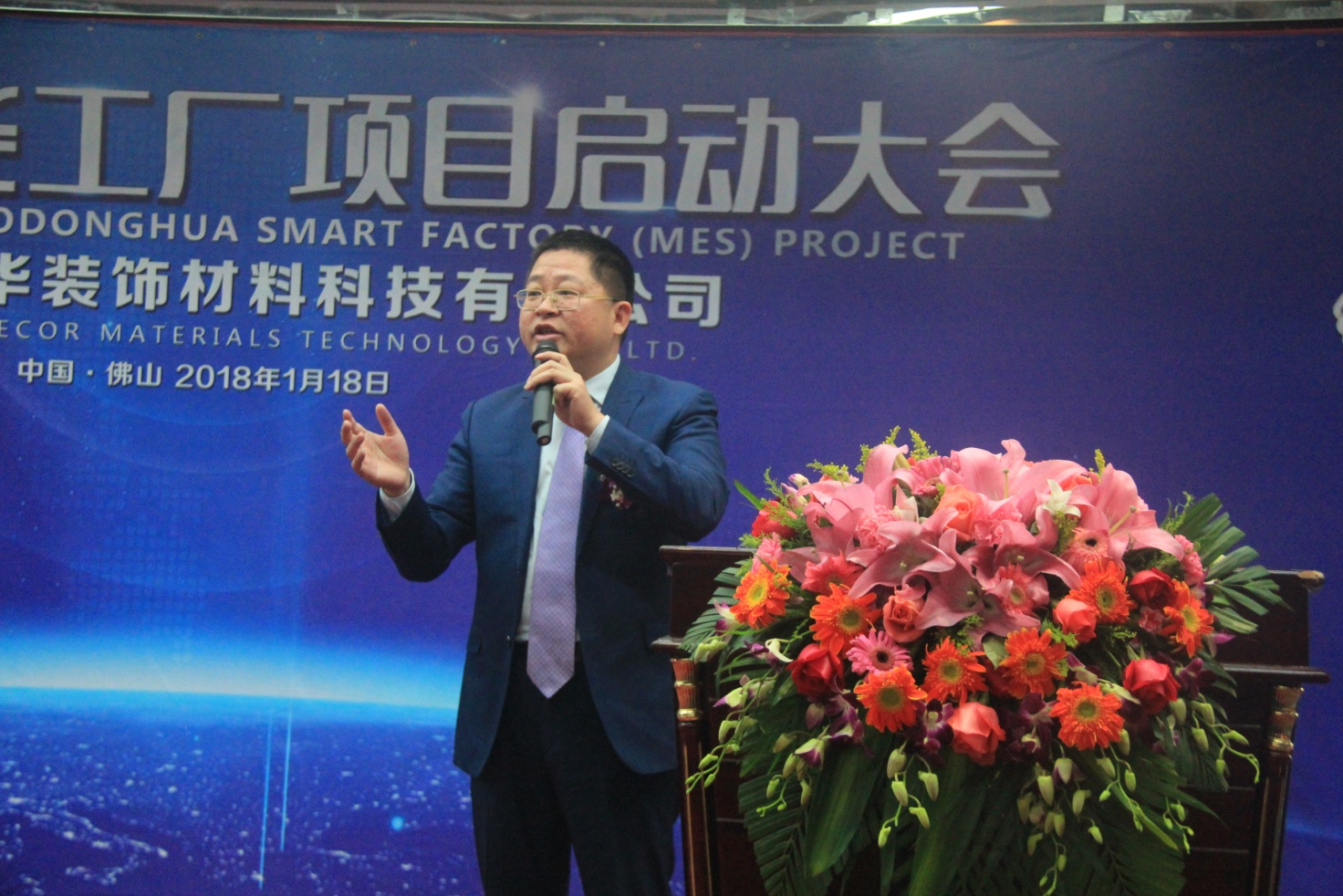速威董事长郭孔快致辞

速威董事长郭孔快表示，MES智能工厂中控系统的启动，彻底解决了传统生产中所存在的手工排产、人工分析设备信息、仓库出货管理不完善、物料信息滞后等弊端，希望耀东华能够发挥行业示范和领头作用，成为世界智能制造标杆企业。速威智能致力于提供工业4.0整体解决方案的专家，成功为国内外上千家知名制造企业和高校提供整体解决方案，在业界享有高度赞誉，是国内工业4.0（智能工厂与智慧物流）解决方案的领军企业。在进一步计划中，携手用友，冠军联盟，强强联合成立用友智能制造广东研究，为中国制造业提供更专业高效的智造，让智能工厂的落地更高效。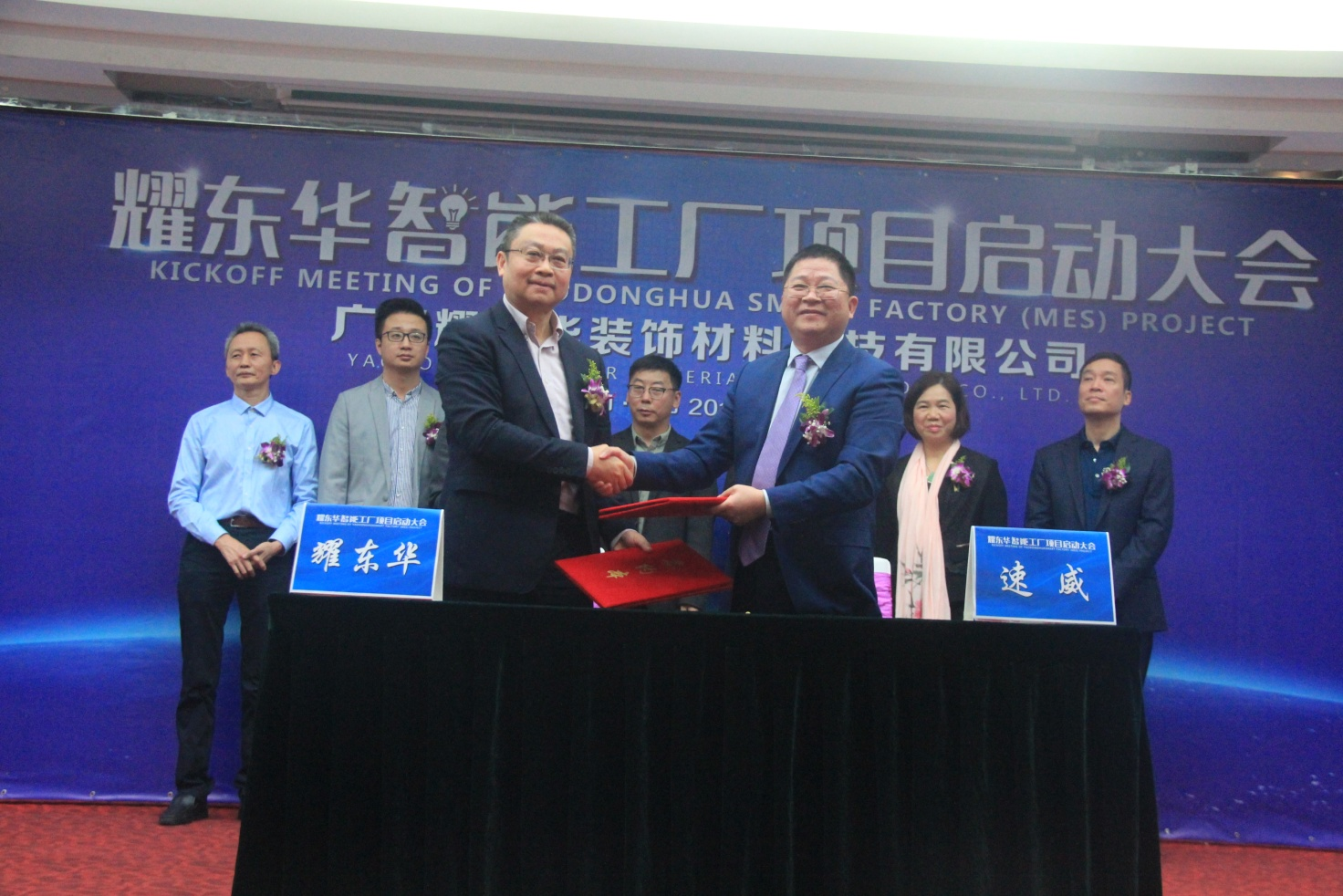签署合作协议

据了解，RTD-MES速威制造执行系统适用于电子、半导体、LED、液晶、触摸屏、空调制造、汽车制造及关键零部件生产、家电、电池、医药等多个行业应用，通过人、机、料、法、环管理生产数据，对生产过程全监控、优化生产调度、实现产品正反追踪追溯、帮助企业实现小批量、多品种生产管理和实现生产透明化，从而降低生产成本和提高生产效率。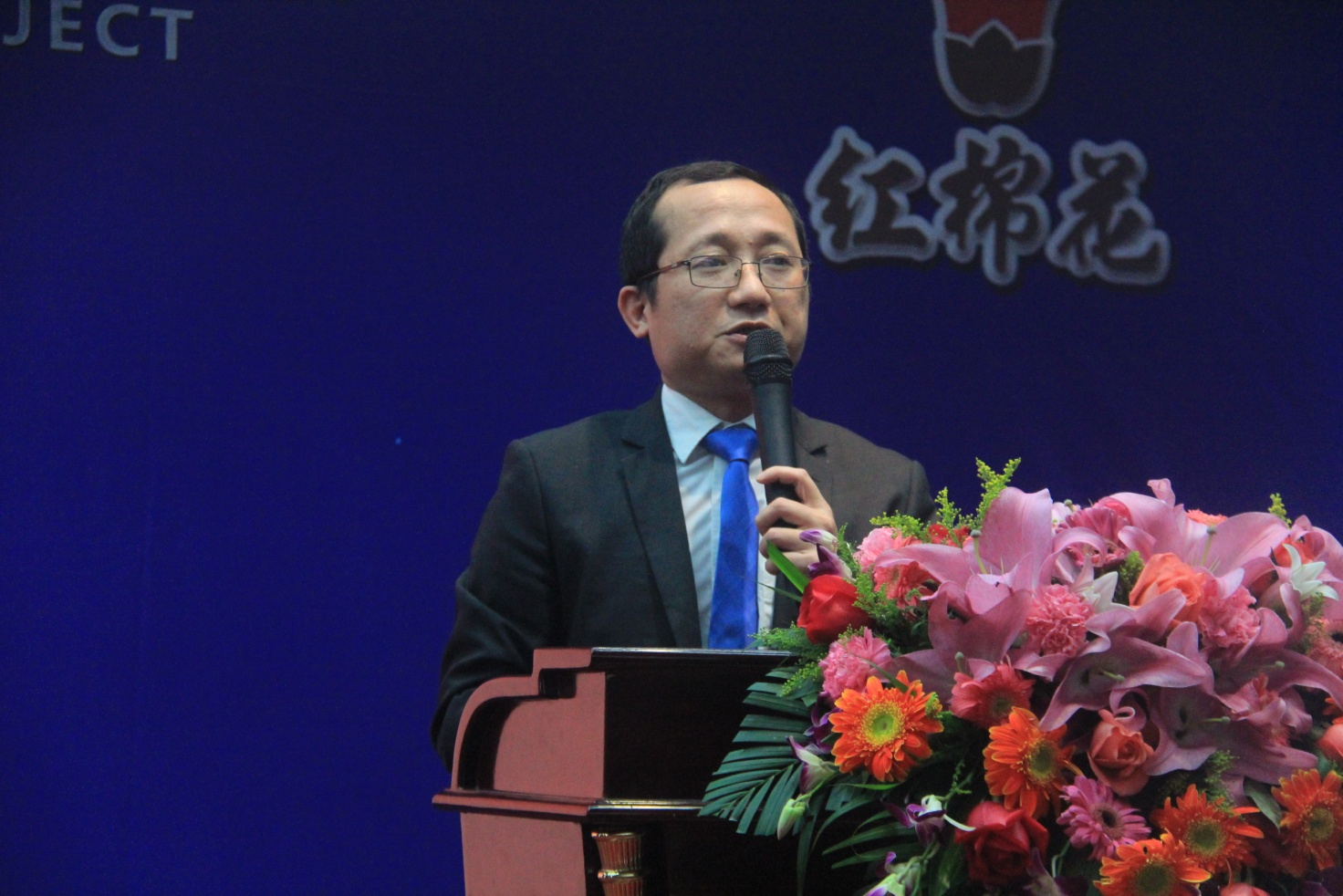广东用友公司制造事业部总经理王浪峰致辞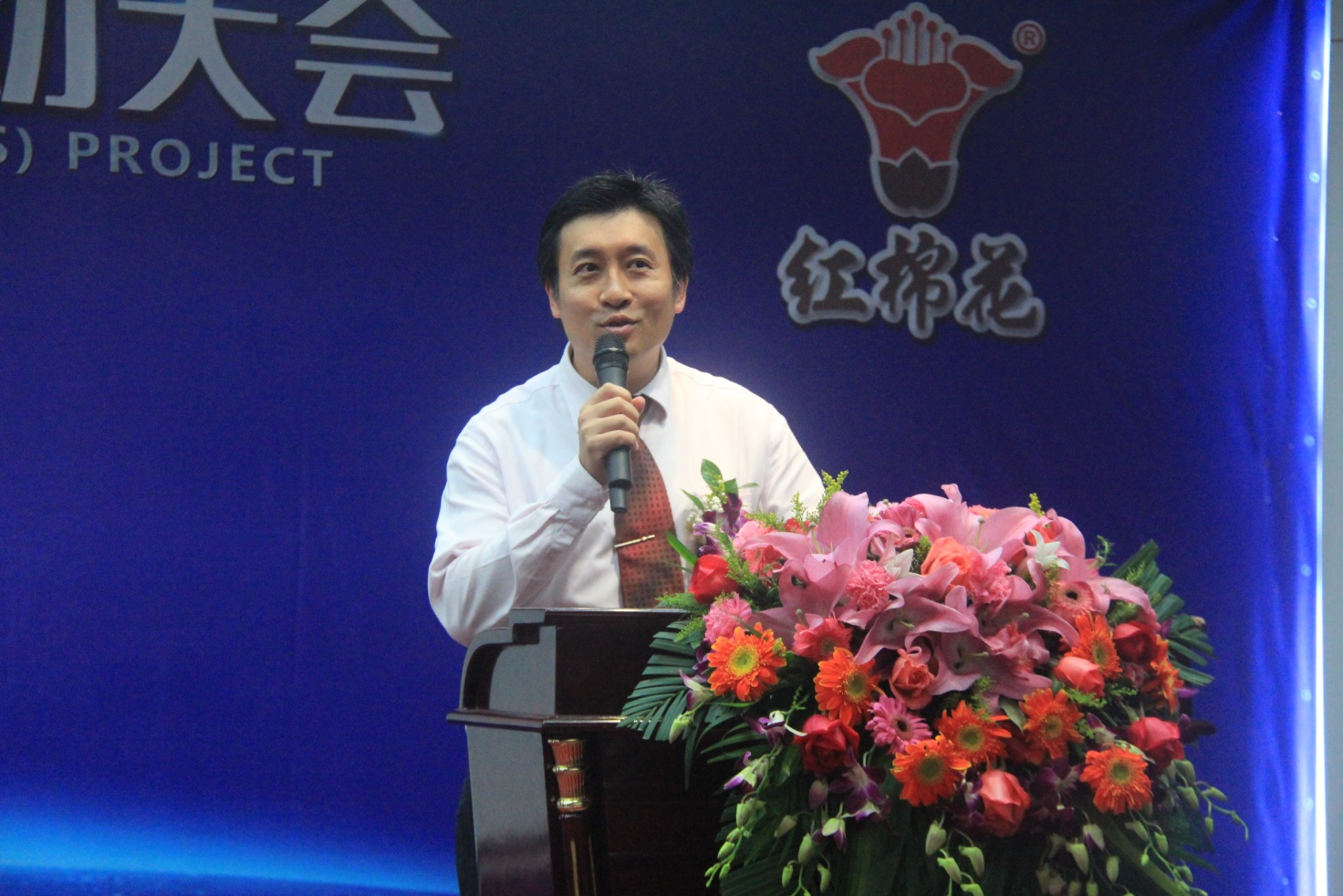速威公司副总经理陈国纬解读耀东华智能工厂项目方案

广州速威智能系统科技有限公司成立于2005年，公司十多年来始终秉承以制造行业与物流行业信息化软件研究、设计、开发、实施为核心，提供智能工厂与智慧物流整体解决方案为主体的专业方向。公司拥有为世界五百强企业进行工业4.0顶层设计咨询专家团队，先进的信息化行业软件研发精英，经验丰富的整体解决方案执行团队，专业的高校教育创新教学师资队伍，拥有百多项发明、实用新型专利、软件著作权。（完）

`声明：本文由入驻焦点开放平台的作者撰写，除焦点官方账号外，观点仅代表作者本人，不代表焦点立场错误信息举报电话： 400-099-0099，邮箱：jubao@vip.sohu.com，或点此进行意见反馈，或点此进行举报投诉。`A B C D E F G H J K L M N P Q R S T W X Y Z
A - B - C - D - E
• A
• 鞍山
• 安庆
• 安阳
• 安顺
• 安康
• 澳门
• B
• 北京
• 保定
• 包头
• 巴彦淖尔
• 本溪
• 蚌埠
• 亳州
• 滨州
• 北海
• 百色
• 巴中
• 毕节
• 保山
• 宝鸡
• 白银
• 巴州
• C
• 承德
• 沧州
• 长治
• 赤峰
• 朝阳
• 长春
• 常州
• 滁州
• 池州
• 长沙
• 常德
• 郴州
• 潮州
• 崇左
• 重庆
• 成都
• 楚雄
• 昌都
• 慈溪
• 常熟
• D
• 大同
• 大连
• 丹东
• 大庆
• 东营
• 德州
• 东莞
• 德阳
• 达州
• 大理
• 德宏
• 定西
• 儋州
• 东平
• E
• 鄂尔多斯
• 鄂州
• 恩施
F - G - H - I - J
• F
• 抚顺
• 阜新
• 阜阳
• 福州
• 抚州
• 佛山
• 防城港
• G
• 赣州
• 广州
• 桂林
• 贵港
• 广元
• 广安
• 贵阳
• 固原
• H
• 邯郸
• 衡水
• 呼和浩特
• 呼伦贝尔
• 葫芦岛
• 哈尔滨
• 黑河
• 淮安
• 杭州
• 湖州
• 合肥
• 淮南
• 淮北
• 黄山
• 菏泽
• 鹤壁
• 黄石
• 黄冈
• 衡阳
• 怀化
• 惠州
• 河源
• 贺州
• 河池
• 海口
• 红河
• 汉中
• 海东
• I
• J
• 晋中
• 锦州
• 吉林
• 鸡西
• 佳木斯
• 嘉兴
• 金华
• 景德镇
• 九江
• 吉安
• 济南
• 济宁
• 焦作
• 荆门
• 荆州
• 江门
• 揭阳
• 金昌
• 酒泉
• 嘉峪关
K - L - M - N - P
• K
• 开封
• 昆明
• 昆山
• L
• 廊坊
• 临汾
• 辽阳
• 连云港
• 丽水
• 六安
• 龙岩
• 莱芜
• 临沂
• 聊城
• 洛阳
• 漯河
• 娄底
• 柳州
• 来宾
• 泸州
• 乐山
• 六盘水
• 丽江
• 临沧
• 拉萨
• 林芝
• 兰州
• 陇南
• M
• 牡丹江
• 马鞍山
• 茂名
• 梅州
• 绵阳
• 眉山
• N
• 南京
• 南通
• 宁波
• 南平
• 宁德
• 南昌
• 南阳
• 南宁
• 内江
• 南充
• P
• 盘锦
• 莆田
• 平顶山
• 濮阳
• 攀枝花
• 普洱
• 平凉
Q - R - S - T - W
• Q
• 秦皇岛
• 齐齐哈尔
• 衢州
• 泉州
• 青岛
• 清远
• 钦州
• 黔南
• 曲靖
• 庆阳
• R
• 日照
• 日喀则
• S
• 石家庄
• 沈阳
• 双鸭山
• 绥化
• 上海
• 苏州
• 宿迁
• 绍兴
• 宿州
• 三明
• 上饶
• 三门峡
• 商丘
• 十堰
• 随州
• 邵阳
• 韶关
• 深圳
• 汕头
• 汕尾
• 三亚
• 三沙
• 遂宁
• 山南
• 商洛
• 石嘴山
• T
• 天津
• 唐山
• 太原
• 通辽
• 铁岭
• 泰州
• 台州
• 铜陵
• 泰安
• 铜仁
• 铜川
• 天水
• 天门
• W
• 乌海
• 乌兰察布
• 无锡
• 温州
• 芜湖
• 潍坊
• 威海
• 武汉
• 梧州
• 渭南
• 武威
• 吴忠
• 乌鲁木齐
X - Y - Z
• X
• 邢台
• 徐州
• 宣城
• 厦门
• 新乡
• 许昌
• 信阳
• 襄阳
• 孝感
• 咸宁
• 湘潭
• 湘西
• 西双版纳
• 西安
• 咸阳
• 西宁
• 仙桃
• 西昌
• Y
• 运城
• 营口
• 盐城
• 扬州
• 鹰潭
• 宜春
• 烟台
• 宜昌
• 岳阳
• 益阳
• 永州
• 阳江
• 云浮
• 玉林
• 宜宾
• 雅安
• 玉溪
• 延安
• 榆林
• 银川
• Z
• 张家口
• 镇江
• 舟山
• 漳州
• 淄博
• 枣庄
• 郑州
• 周口
• 驻马店
• 株洲
• 张家界
• 珠海
• 湛江
• 肇庆
• 中山
• 自贡
• 资阳
• 遵义
• 昭通
• 张掖
• 中卫

1室1厅1厨1卫1阳台

1
2
3
4
5

0
1
2

1

1

0
1
2
3报名成功，资料已提交审核A B C D E F G H J K L M N P Q R S T W X Y Z
A - B - C - D - E
• A
• 鞍山
• 安庆
• 安阳
• 安顺
• 安康
• 澳门
• B
• 北京
• 保定
• 包头
• 巴彦淖尔
• 本溪
• 蚌埠
• 亳州
• 滨州
• 北海
• 百色
• 巴中
• 毕节
• 保山
• 宝鸡
• 白银
• 巴州
• C
• 承德
• 沧州
• 长治
• 赤峰
• 朝阳
• 长春
• 常州
• 滁州
• 池州
• 长沙
• 常德
• 郴州
• 潮州
• 崇左
• 重庆
• 成都
• 楚雄
• 昌都
• 慈溪
• 常熟
• D
• 大同
• 大连
• 丹东
• 大庆
• 东营
• 德州
• 东莞
• 德阳
• 达州
• 大理
• 德宏
• 定西
• 儋州
• 东平
• E
• 鄂尔多斯
• 鄂州
• 恩施
F - G - H - I - J
• F
• 抚顺
• 阜新
• 阜阳
• 福州
• 抚州
• 佛山
• 防城港
• G
• 赣州
• 广州
• 桂林
• 贵港
• 广元
• 广安
• 贵阳
• 固原
• H
• 邯郸
• 衡水
• 呼和浩特
• 呼伦贝尔
• 葫芦岛
• 哈尔滨
• 黑河
• 淮安
• 杭州
• 湖州
• 合肥
• 淮南
• 淮北
• 黄山
• 菏泽
• 鹤壁
• 黄石
• 黄冈
• 衡阳
• 怀化
• 惠州
• 河源
• 贺州
• 河池
• 海口
• 红河
• 汉中
• 海东
• I
• J
• 晋中
• 锦州
• 吉林
• 鸡西
• 佳木斯
• 嘉兴
• 金华
• 景德镇
• 九江
• 吉安
• 济南
• 济宁
• 焦作
• 荆门
• 荆州
• 江门
• 揭阳
• 金昌
• 酒泉
• 嘉峪关
K - L - M - N - P
• K
• 开封
• 昆明
• 昆山
• L
• 廊坊
• 临汾
• 辽阳
• 连云港
• 丽水
• 六安
• 龙岩
• 莱芜
• 临沂
• 聊城
• 洛阳
• 漯河
• 娄底
• 柳州
• 来宾
• 泸州
• 乐山
• 六盘水
• 丽江
• 临沧
• 拉萨
• 林芝
• 兰州
• 陇南
• M
• 牡丹江
• 马鞍山
• 茂名
• 梅州
• 绵阳
• 眉山
• N
• 南京
• 南通
• 宁波
• 南平
• 宁德
• 南昌
• 南阳
• 南宁
• 内江
• 南充
• P
• 盘锦
• 莆田
• 平顶山
• 濮阳
• 攀枝花
• 普洱
• 平凉
Q - R - S - T - W
• Q
• 秦皇岛
• 齐齐哈尔
• 衢州
• 泉州
• 青岛
• 清远
• 钦州
• 黔南
• 曲靖
• 庆阳
• R
• 日照
• 日喀则
• S
• 石家庄
• 沈阳
• 双鸭山
• 绥化
• 上海
• 苏州
• 宿迁
• 绍兴
• 宿州
• 三明
• 上饶
• 三门峡
• 商丘
• 十堰
• 随州
• 邵阳
• 韶关
• 深圳
• 汕头
• 汕尾
• 三亚
• 三沙
• 遂宁
• 山南
• 商洛
• 石嘴山
• T
• 天津
• 唐山
• 太原
• 通辽
• 铁岭
• 泰州
• 台州
• 铜陵
• 泰安
• 铜仁
• 铜川
• 天水
• 天门
• W
• 乌海
• 乌兰察布
• 无锡
• 温州
• 芜湖
• 潍坊
• 威海
• 武汉
• 梧州
• 渭南
• 武威
• 吴忠
• 乌鲁木齐
X - Y - Z
• X
• 邢台
• 徐州
• 宣城
• 厦门
• 新乡
• 许昌
• 信阳
• 襄阳
• 孝感
• 咸宁
• 湘潭
• 湘西
• 西双版纳
• 西安
• 咸阳
• 西宁
• 仙桃
• 西昌
• Y
• 运城
• 营口
• 盐城
• 扬州
• 鹰潭
• 宜春
• 烟台
• 宜昌
• 岳阳
• 益阳
• 永州
• 阳江
• 云浮
• 玉林
• 宜宾
• 雅安
• 玉溪
• 延安
• 榆林
• 银川
• Z
• 张家口
• 镇江
• 舟山
• 漳州
• 淄博
• 枣庄
• 郑州
• 周口
• 驻马店
• 株洲
• 张家界
• 珠海
• 湛江
• 肇庆
• 中山
• 自贡
• 资阳
• 遵义
• 昭通
• 张掖
• 中卫• 手机• 分享
• 设计
免费设计
• 计算器
装修计算器
• 入驻
合作入驻
• 联系
联系我们
• 置顶
返回顶部